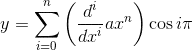# General Solution to a certain form of ODE

While fiddling around with some very simple linear ODEs, I "discovered" a formula that gives a solution to ODEs of the form: ##y'+y=ax^n ##.

here it is:i'm sure that this was discovered before, but i was just wondering if it had any official name or something.

You can find the general solution to this equation by using an integrating factor...

$y= a e^{-x}\int{ e^x x^n dx}+ Ce^{-x}$

The integral can be expressed in terms of the incomplete gamma function.
For positive integer $n$ it simplifies to
$\int{ e^x x^n dx}=e^x\left(x^n - n x^{n-1}+n\left(n-1\right)x^{n-2}\dots -1^n n! \right)$

This will reproduce your solution for the case $C=0$ .

yes, that's how I derived it. I was just wondering if it had a name.

Astronuc
Staff Emeritus
yes, that's how I derived it. I was just wondering if it had a name.

The equation in the OP is a first order linear ordinary differential equation of the general form y' + p(x) y = q(x), with p(x) = 1, q(x) = axn.
http://tutorial.math.lamar.edu/Classes/DE/Linear.aspx

http://mathworld.wolfram.com/First-OrderOrdinaryDifferentialEquation.html

There does not appear to be a special name for that particular form. Perhaps there was way back when.

Some named first and second order ordinary differential equations found here:
http://mathworld.wolfram.com/OrdinaryDifferentialEquation.html

Last edited: